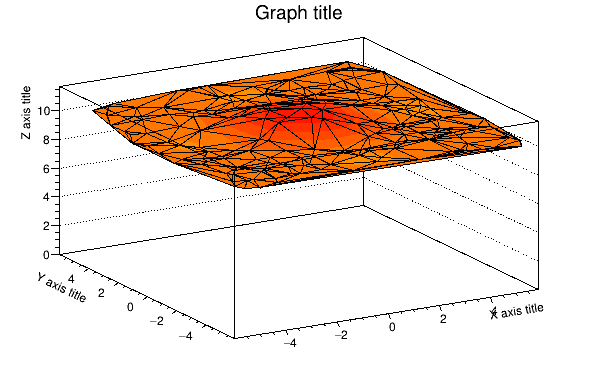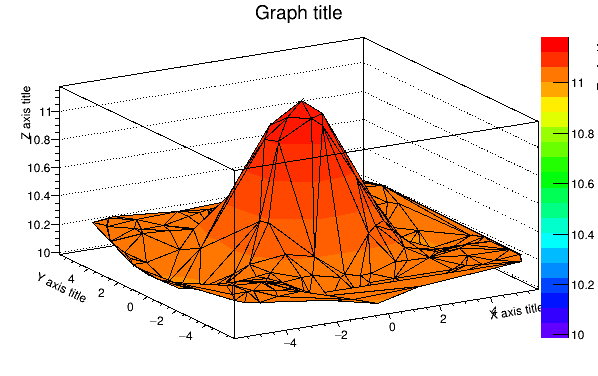# TGraph2D "TRI1" option color range

I am trying to draw a TGraph2D with the “TRI1” drawing option containing data with a z range that is significantly offset from zero.

Here is a slight modification of the SURF1 example given in the TGraph2D class reference:

``````{
auto c = new TCanvas("c","Graph2D example",0,0,600,400);
Double_t x, y, z, P = 6.;
Int_t np = 200;
auto dt = new TGraph2D();
dt->SetTitle("Graph title; X axis title; Y axis title; Z axis title");
auto r = new TRandom();
for (Int_t N=0; N<np; N++) {
x = 2*P*(r->Rndm(N))-P;
y = 2*P*(r->Rndm(N))-P;
z = (sin(x)/x)*(sin(y)/y)+0.2 + 10.;
dt->SetPoint(N,x,y,z);
}
gStyle->SetPalette(1);
// Setting z axis range doesn't work.
//   dt->Draw("tri1z");
//   dt->GetZaxis()->SetRangeUser(dt->GetZmin(),
//      				dt->GetZmax());

// Setting z axis range works, but colors are not updated.
auto dth = dt->GetHistogram();
dth->Draw("tri1z");
dth->GetZaxis()->SetRangeUser(dt->GetZmin(),
dt->GetZmax());

}
``````

If I draw the TGraph2D with the “tri1z” option, I find that using `GetZaxis()->SetRangeUser()` to set the z axis range has no effect. The lower limit of the z axis (and the corresponding color map) has a lower limit of 0:If I use the GetHistogram() method and try drawing the histogram instead, setting the z axis range does work, and the color range bar updates, but the coloring of the surface is not updated accordingly.ROOT Version: 6.28/04
Platform: linuxx8664gcc
Compiler: c++ (Ubuntu 9.4.0-1ubuntu1~20.04.1) 9.4.0

1 Like

Hi,

``````   dt->SetMinimum(dt->GetZmin() - 0.1);